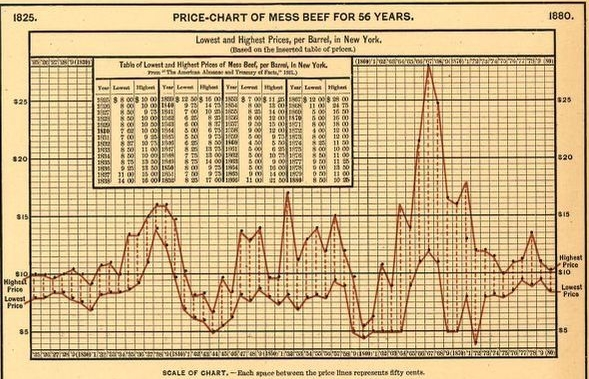# Teaching Mathematics Using Primary Sources: Data Mining, 1880s Style, Part 2

This post was written by Peter DeCraene, the 2020-21 Albert Einstein Distinguished Educator Fellow at the Library of Congress. This is one of several posts about using mathematics to develop and analyze data representations found in primary sources.

Statistical atlases contain a wealth of data and data representations that can be used to connect mathematics topics to U.S. History. A page from Scribner’s Statistical Atlas of the United States – published in 1883 using data from the 1880 Census – contains four different ways to represent data. An earlier post looked at tables, bar graphs, and a map from this page; this time, we’ll focus on the lower half of the page, with a different table and a graph showing trends over time.

The data here is about the price of “Mess Beef.” (I had to look that up.) This graph contains more information than students might typically see on a graph in a textbook. To help students analyze the information, start with the Observe-Reflect-Question technique. This low-threshold activity (also referred to as “Notice and Wonder” by some math teachers) allows all students to join the discussion and has a high ceiling for what can be done from there.Price Chart for Mess Beef for 56 Years from Scribner’s statistical atlas of the United States, 1883

Ask students to spend a few minutes making notes about what they observe or notice about the information, and share their ideas with group-mates. Next, invite groups to write any questions they have about what they see – what do they wonder about the data? Working as a class, sort questions into two groups: questions that may need more information to answer, and questions that can be answered mathematically. The first group could lead to some interesting social studies lessons about economics: What was the consumer cost for beef at this time? How did that compare to the wages earned?

Questions about functions and trends can be used to help students interpret this type of graph mathematically:

• In what year(s) was the price of beef the highest? (This question could also lead to a history question: what was going on in the country in the years when the prices of beef spiked?)
• In what year(s) was the price lowest?  (This should cause some discussion, because the lowest minimum price occurred in 1872, but the lowest maximum price was in 1860.)
• Where is the difference between the maximum and minimum prices the smallest? (In my experience, students will sometimes talk about the two dots representing 1840 and 1841 being very close together, forgetting that the range of values would be represented by the vertical dashed lines.)
• In an algebra class, you might ask: “Does this graph represent a function?”

Some questions require deeper mathematical analysis: What was the average price of beef between 1825 and 1880? There are several reasonable methods students might use to find an average, and different methods may result in different average values. For a different way to represent the data, graph the trend in the differences between the maximum and minimum prices in terms of the year; what might that representation reveal? Ask: Based on any of the information you have, would you say the price of beef was generally trending upward or downward during this period? Creating a spreadsheet to explore some of these questions and to find a trend line might lead to other questions. Allow time for students to explain their thinking and the choices they made as they solved these problems.

This type of graph might also serve as an example for students to create their own data representations of other trends of their choosing. What questions and representations do your students come up with? If you look further at this Statistical Atlas, what other diagrams and graphs might you use in your classroom? Please take a moment to comment below.